# 火锅底料产品

•我要代理
•我要代理
•我要代理
•我要代理
•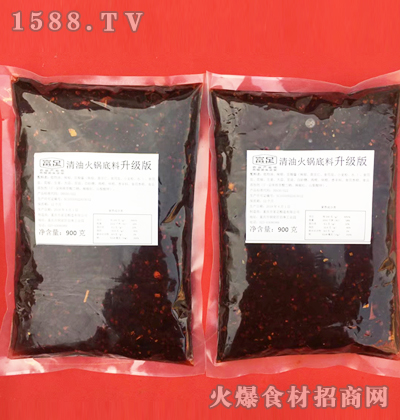我要代理
•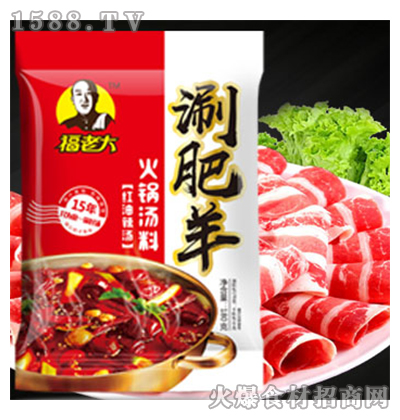我要代理
•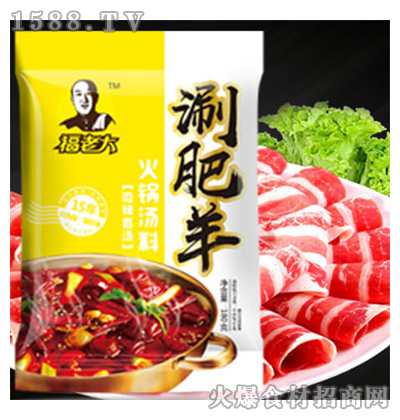我要代理
•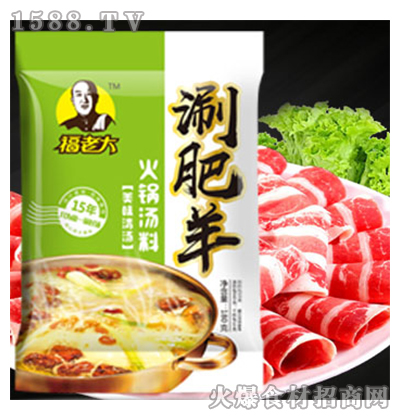我要代理
•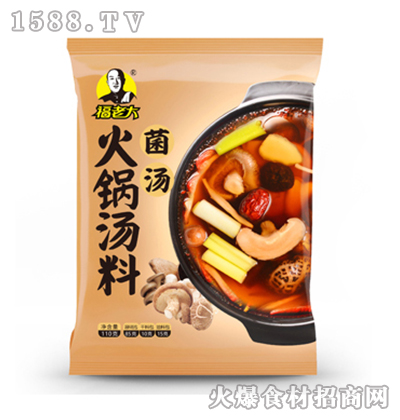我要代理
•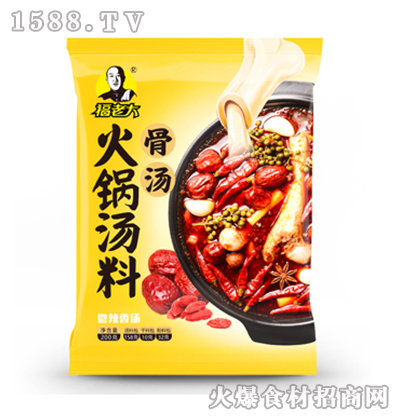我要代理
•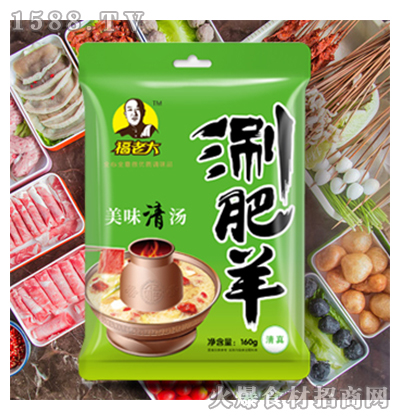我要代理
•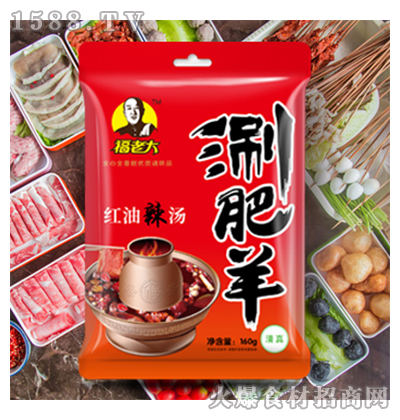我要代理
•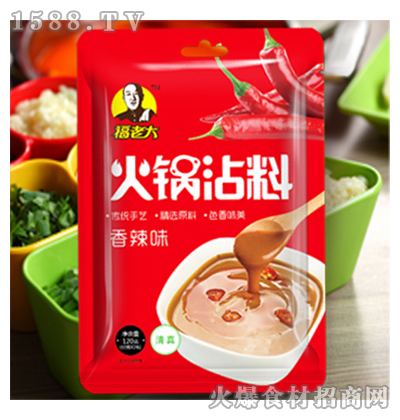我要代理
•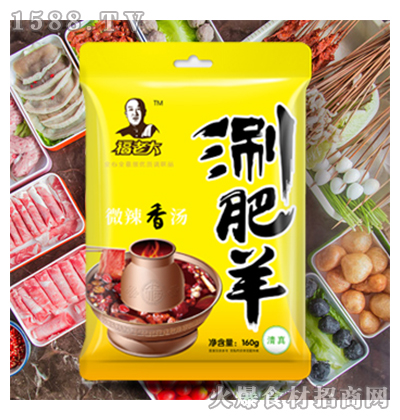我要代理
•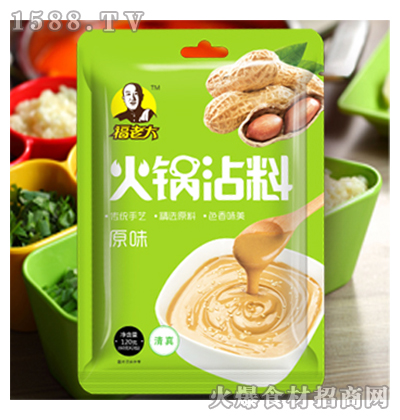我要代理
•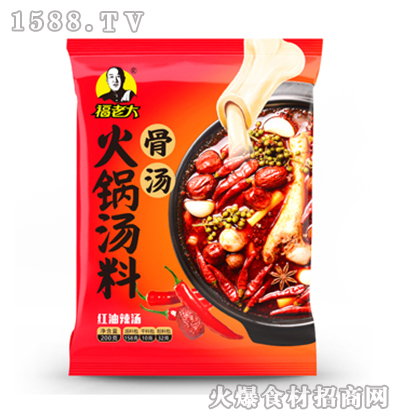我要代理
•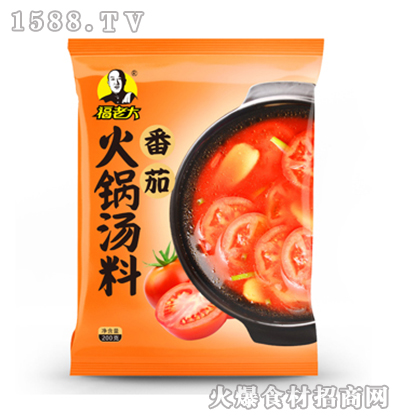我要代理
•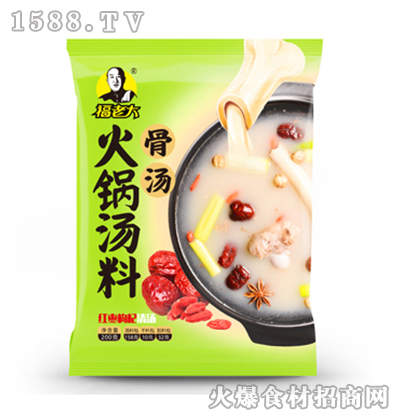我要代理
•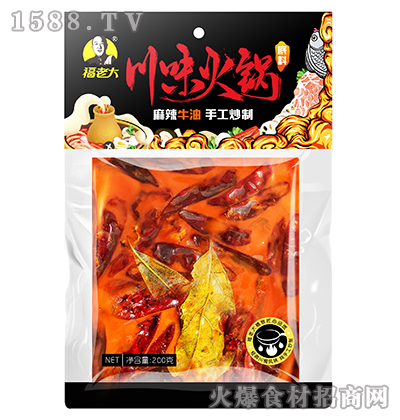我要代理
•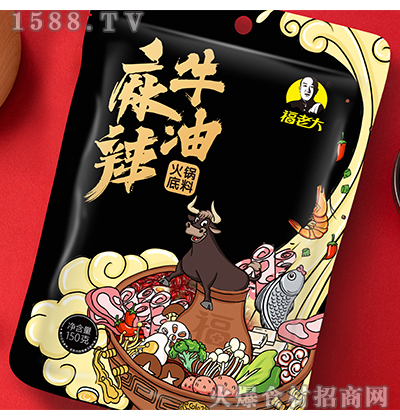我要代理
•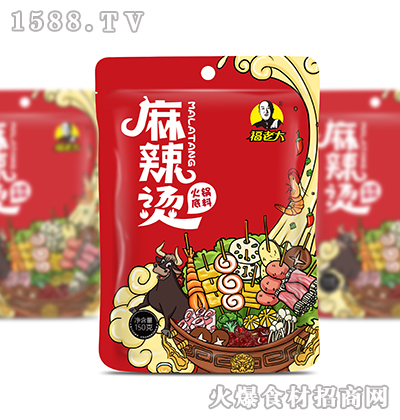我要代理
•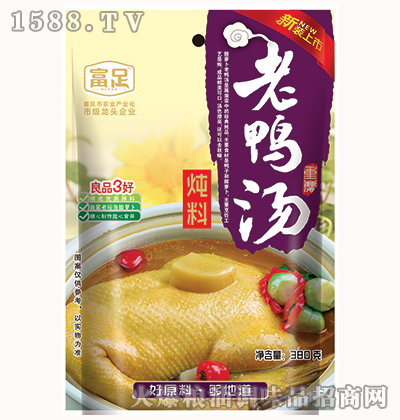我要代理
•我要代理
•我要代理
•我要代理
•我要代理
•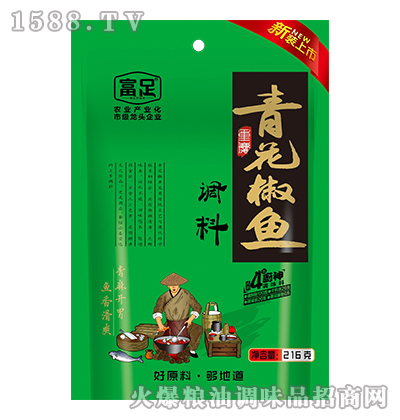我要代理
•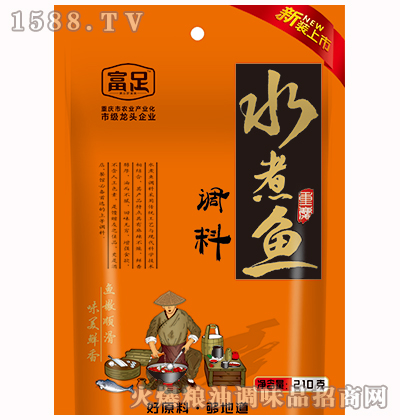我要代理
•我要代理
•我要代理
•我要代理
•我要代理
•我要代理
•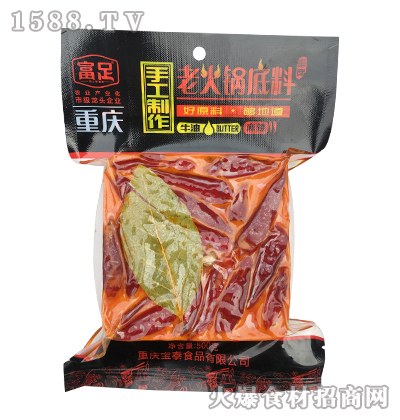我要代理
•我要代理
•我要代理
•我要代理
•我要代理
•我要代理
•我要代理
•我要代理
•我要代理
•我要代理
•我要代理
•我要代理
•我要代理
•我要代理
•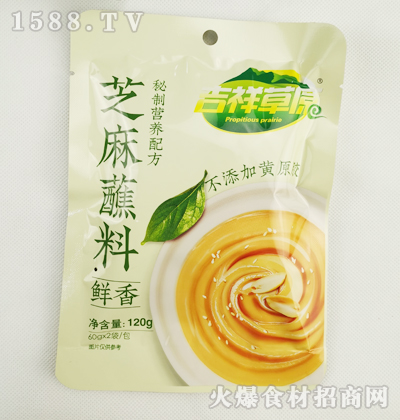我要代理
•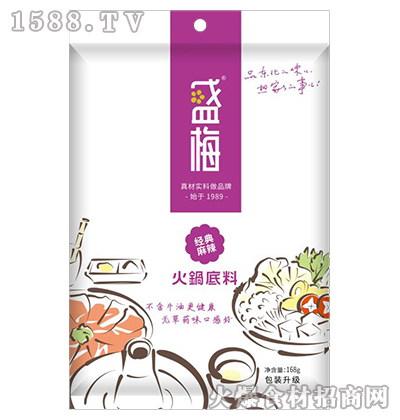我要代理
•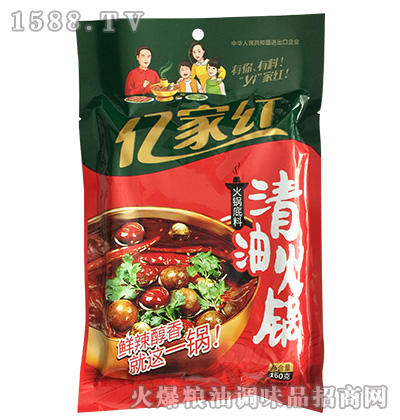我要代理
•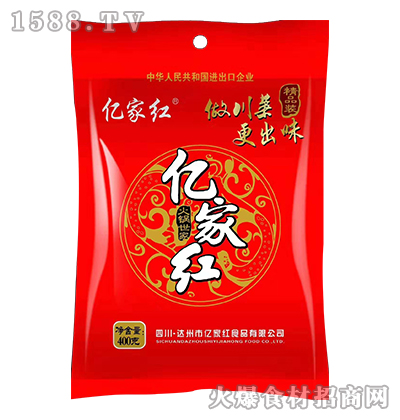我要代理
•我要代理
•我要代理
•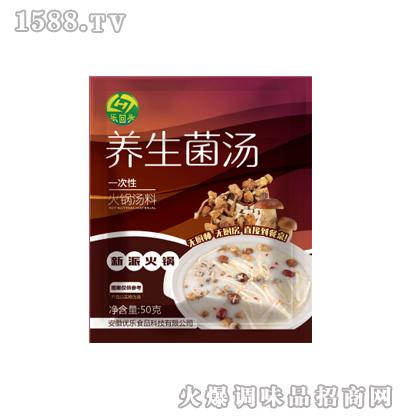我要代理
•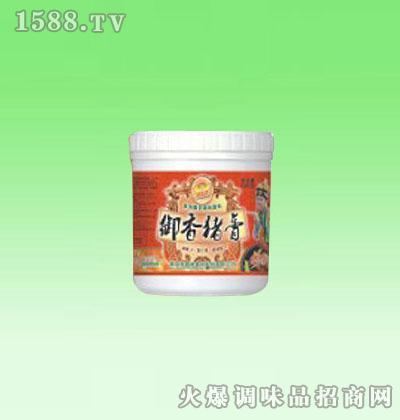我要代理
•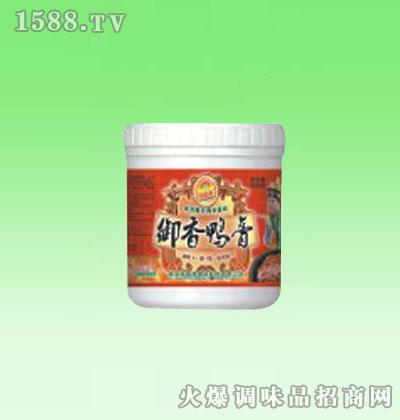我要代理
•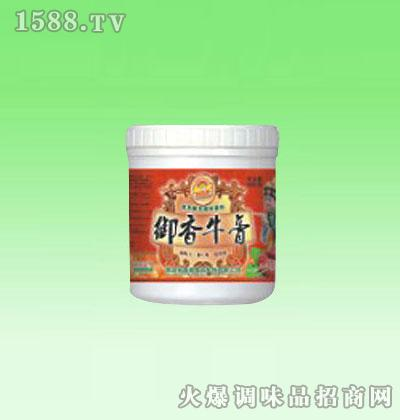我要代理
•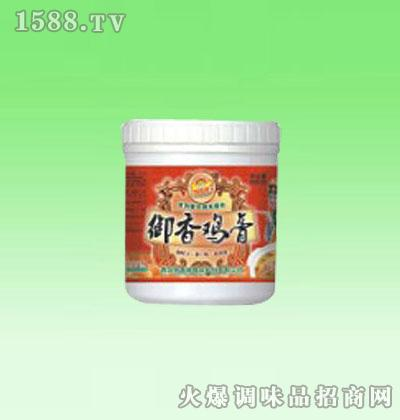我要代理
•我要代理
•我要代理
•我要代理
•我要代理
•我要代理
•我要代理
•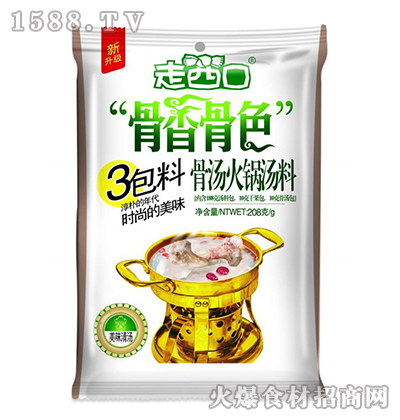我要代理
•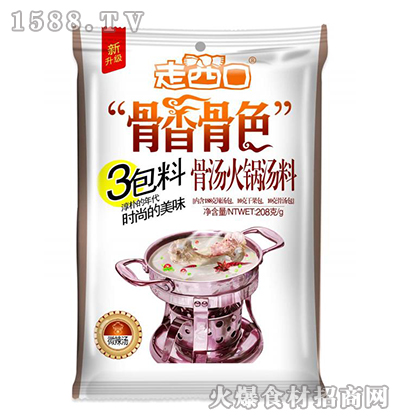我要代理
•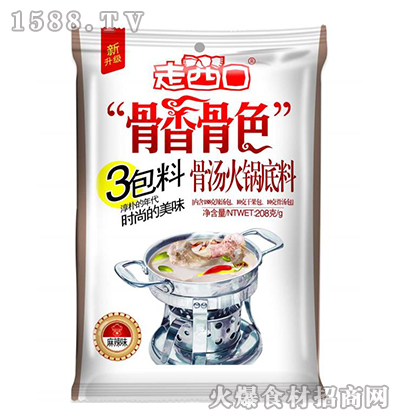我要代理
•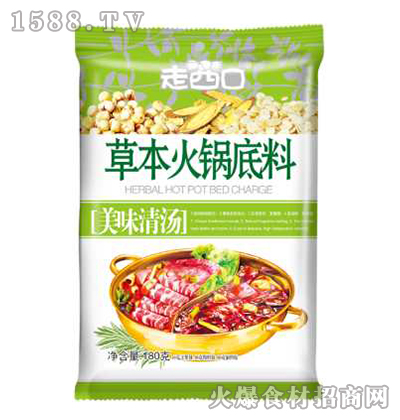我要代理
•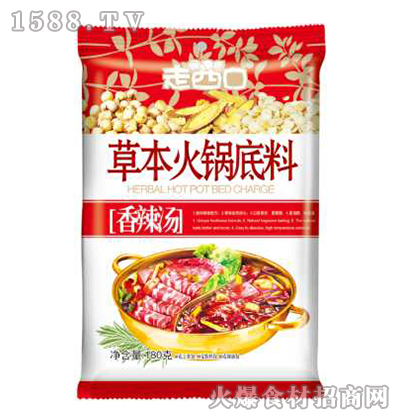我要代理
•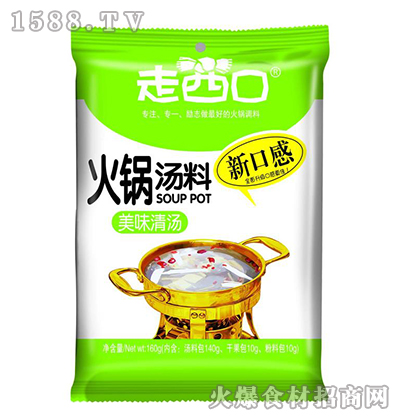我要代理